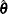#### Logistic Regression Models

If the response categories of the response variable Y can be restricted to a number of ordinal values, you can fit cumulative probabilities of the response categories with a cumulative logit model, a complementary log-log model, or a probit model. Details of cumulative logit models (or proportional odds models) can be found in McCullagh and Nelder (1989). If the response categories of Y are nominal responses without natural ordering, you can fit the response probabilities with a generalized logit model. Formulation of the generalized logit models for nominal response variables can be found in Agresti (2002). For each model, the procedure estimates the model parameterby using a pseudo-log-likelihood function. The procedure obtains the pseudo-maximum likelihood estimatorby using iterations described in the section Iterative Algorithms for Model Fitting and estimates its variance described in the section Variance Estimation.